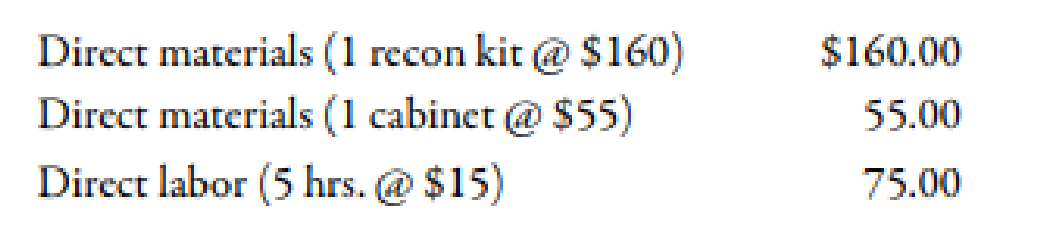Chapter 10, Problem 43E### Managerial Accounting: The Corners...

7th Edition
Maryanne M. Mowen + 2 others
ISBN: 9781337115773

#### Solutions

Chapter
Section### Managerial Accounting: The Corners...

7th Edition
Maryanne M. Mowen + 2 others
ISBN: 9781337115773
Textbook Problem
23 views

# Standard Quantities of Labor and MaterialsStillwater Designs rebuilds defective units of its S12L7 Kicker speaker model. During the year, Stillwater rebuilt 15,000 units. Materials and labor standards for performing the repairs are as follows:Required:Compute the standard hours allowed for a volume of 15,000 rebuilt units.Compute the standard number of kits and cabinets allowed for a volume of 15,000 rebuilt units.Suppose that during the first month of the year, 15,000 standard hours were allowed for the units rebuilt. How many units were rebuilt during the first month?

1.

To determine

Calculate the value of standard hours for a volume of 15,000 rebuilt units.

Explanation

Standard Costing:

Standard costing is an accounting tool which is used to measure the variances in the cost.

The amount obtained when actual cost is deducted from budgeted cost is known as variance. Variance is calculated to find whether the cost is over applied or under applied.

Use the following formula to calculate the value of standard hours:

Standard Hours=Actual Units×Direct

2.

To determine

Calculate the standard quantity of kits and cabinets for a volume of 15,000 units.

3.

To determine

Calculate the amount of units produced during the first month.

### Still sussing out bartleby?

Check out a sample textbook solution.

See a sample solution

#### The Solution to Your Study Problems

Bartleby provides explanations to thousands of textbook problems written by our experts, many with advanced degrees!

Get Started

#### Find more solutions based on key concepts